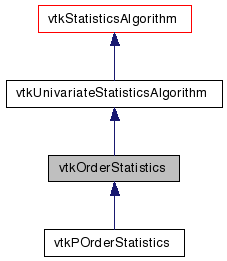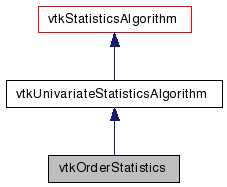# vtkOrderStatistics Class Reference

`#include <vtkOrderStatistics.h>`

Inheritance diagram for vtkOrderStatistics:[legend]
Collaboration diagram for vtkOrderStatistics:[legend]

## Detailed Description

A class for univariate order statistics.

Given a selection of columns of interest in an input data table, this class provides the following functionalities, depending on the execution mode it is executed in: Learn: calculate arbitrary quantiles. Provide specific names when 5-point statistics (minimum, 1st quartile, median, third quartile, maximum) requested. Assess: given an input data set and a set of q-quantiles, label each datum either with the quantile interval to which it belongs, or 0 if it is smaller than smaller quantile, or q if it is larger than largest quantile.

Thanks:
Thanks to Philippe Pebay and David Thompson from Sandia National Laboratories for implementing this class.
Examples:
vtkOrderStatistics (Examples)
Tests:
vtkOrderStatistics (Tests)

Definition at line 54 of file vtkOrderStatistics.h.

## Public Types

typedef
vtkUnivariateStatisticsAlgorithm
Superclass
enum  QuantileDefinitionType { InverseCDF = 0, InverseCDFAveragedSteps = 1 }

## Public Member Functions

virtual const char * GetClassName ()
virtual int IsA (const char *type)
void PrintSelf (ostream &os, vtkIndent indent)
vtkIdType GetQuantileDefinition ()
virtual void SetNumericType (int)
virtual int GetNumericType ()
virtual void NumericTypeOn ()
virtual void NumericTypeOff ()
virtual void SetNumberOfIntervals (vtkIdType)
virtual vtkIdType GetNumberOfIntervals ()
virtual void SetQuantileDefinition (QuantileDefinitionType)
void SetQuantileDefinition (int)
virtual bool SetParameter (const char *parameter, int index, vtkVariant value)
virtual void Aggregate (vtkDataObjectCollection *, vtkMultiBlockDataSet *)

## Static Public Member Functions

static int IsTypeOf (const char *type)
static vtkOrderStatisticsSafeDownCast (vtkObject *o)
static vtkOrderStatisticsNew ()

## Protected Member Functions

vtkOrderStatistics ()
~vtkOrderStatistics ()
virtual void Derive (vtkMultiBlockDataSet *)
virtual void Learn (vtkTable *inData, vtkTable *inParameters, vtkMultiBlockDataSet *outMeta)
virtual void Test (vtkTable *, vtkMultiBlockDataSet *, vtkTable *)
virtual void SelectAssessFunctor (vtkTable *outData, vtkDataObject *inMeta, vtkStringArray *rowNames, AssessFunctor *&dfunc)

## Protected Attributes

int NumericType
int NumberOfIntervals
QuantileDefinitionType QuantileDefinition

## Member Typedef Documentation

Reimplemented from vtkUnivariateStatisticsAlgorithm.

Reimplemented in vtkPOrderStatistics.

Definition at line 57 of file vtkOrderStatistics.h.

## Member Enumeration Documentation

The type of quantile definition.

Enumerator:
 InverseCDF InverseCDFAveragedSteps

Definition at line 64 of file vtkOrderStatistics.h.

## Constructor & Destructor Documentation

 vtkOrderStatistics::vtkOrderStatistics ( ) ` [protected]`

 vtkOrderStatistics::~vtkOrderStatistics ( ) ` [protected]`

## Member Function Documentation

 virtual const char* vtkOrderStatistics::GetClassName ( ) ` [virtual]`

Reimplemented from vtkUnivariateStatisticsAlgorithm.

Reimplemented in vtkPOrderStatistics.

 static int vtkOrderStatistics::IsTypeOf ( const char * name ) ` [static]`

Return 1 if this class type is the same type of (or a subclass of) the named class. Returns 0 otherwise. This method works in combination with vtkTypeMacro found in vtkSetGet.h.

Reimplemented from vtkUnivariateStatisticsAlgorithm.

Reimplemented in vtkPOrderStatistics.

 virtual int vtkOrderStatistics::IsA ( const char * name ) ` [virtual]`

Return 1 if this class is the same type of (or a subclass of) the named class. Returns 0 otherwise. This method works in combination with vtkTypeMacro found in vtkSetGet.h.

Reimplemented from vtkUnivariateStatisticsAlgorithm.

Reimplemented in vtkPOrderStatistics.

 static vtkOrderStatistics* vtkOrderStatistics::SafeDownCast ( vtkObject * o ) ` [static]`

Reimplemented from vtkUnivariateStatisticsAlgorithm.

Reimplemented in vtkPOrderStatistics.

 void vtkOrderStatistics::PrintSelf ( ostream & os, vtkIndent indent ) ` [virtual]`

Methods invoked by print to print information about the object including superclasses. Typically not called by the user (use Print() instead) but used in the hierarchical print process to combine the output of several classes.

Reimplemented from vtkUnivariateStatisticsAlgorithm.

Reimplemented in vtkPOrderStatistics.

 static vtkOrderStatistics* vtkOrderStatistics::New ( ) ` [static]`

Create an object with Debug turned off, modified time initialized to zero, and reference counting on.

Reimplemented from vtkTableAlgorithm.

Reimplemented in vtkPOrderStatistics.

 virtual void vtkOrderStatistics::SetNumericType ( int ) ` [virtual]`

Set/get whether histograms and assessment data should be treated as numeric data. Otherwise, then everything is treated as strings, which always works, and is thus the the default. Note that if the data is indeed numeric but this is not set, some strange results will occur because of the use of the lexicographic order instead of the order on reals.

 virtual int vtkOrderStatistics::GetNumericType ( ) ` [virtual]`

Set/get whether histograms and assessment data should be treated as numeric data. Otherwise, then everything is treated as strings, which always works, and is thus the the default. Note that if the data is indeed numeric but this is not set, some strange results will occur because of the use of the lexicographic order instead of the order on reals.

 virtual void vtkOrderStatistics::NumericTypeOn ( ) ` [virtual]`

Set/get whether histograms and assessment data should be treated as numeric data. Otherwise, then everything is treated as strings, which always works, and is thus the the default. Note that if the data is indeed numeric but this is not set, some strange results will occur because of the use of the lexicographic order instead of the order on reals.

 virtual void vtkOrderStatistics::NumericTypeOff ( ) ` [virtual]`

Set/get whether histograms and assessment data should be treated as numeric data. Otherwise, then everything is treated as strings, which always works, and is thus the the default. Note that if the data is indeed numeric but this is not set, some strange results will occur because of the use of the lexicographic order instead of the order on reals.

 virtual void vtkOrderStatistics::SetNumberOfIntervals ( vtkIdType ) ` [virtual]`

Set/Get the number of quantiles (with uniform spacing).

 virtual vtkIdType vtkOrderStatistics::GetNumberOfIntervals ( ) ` [virtual]`

Set/Get the number of quantiles (with uniform spacing).

 virtual void vtkOrderStatistics::SetQuantileDefinition ( QuantileDefinitionType ) ` [virtual]`

Set the quantile definition.

 void vtkOrderStatistics::SetQuantileDefinition ( int )

Set the quantile definition.

 vtkIdType vtkOrderStatistics::GetQuantileDefinition ( ) ` [inline]`

Get the quantile definition.

Definition at line 96 of file vtkOrderStatistics.h.

 virtual bool vtkOrderStatistics::SetParameter ( const char * parameter, int index, vtkVariant value ) ` [virtual]`

A convenience method (in particular for access from other applications) to set parameter values. Return true if setting of requested parameter name was excuted, false otherwise.

Reimplemented from vtkStatisticsAlgorithm.

 virtual void vtkOrderStatistics::Aggregate ( vtkDataObjectCollection * , vtkMultiBlockDataSet * ) ` [inline, virtual]`

Given a collection of models, calculate aggregate model NB: not implemented

Implements vtkStatisticsAlgorithm.

Definition at line 110 of file vtkOrderStatistics.h.

 virtual void vtkOrderStatistics::Learn ( vtkTable * inData, vtkTable * inParameters, vtkMultiBlockDataSet * outMeta ) ` [protected, virtual]`

Execute the calculations required by the Learn option.

Implements vtkStatisticsAlgorithm.

Reimplemented in vtkPOrderStatistics.

 virtual void vtkOrderStatistics::Derive ( vtkMultiBlockDataSet * ) ` [protected, virtual]`

Execute the calculations required by the Derive option.

Implements vtkStatisticsAlgorithm.

 virtual void vtkOrderStatistics::Test ( vtkTable * , vtkMultiBlockDataSet * , vtkTable * ) ` [protected, virtual]`

Execute the calculations required by the Test option.

Implements vtkStatisticsAlgorithm.

 virtual void vtkOrderStatistics::SelectAssessFunctor ( vtkTable * outData, vtkDataObject * inMeta, vtkStringArray * rowNames, AssessFunctor *& dfunc ) ` [protected, virtual]`

Provide the appropriate assessment functor.

Implements vtkStatisticsAlgorithm.

## Member Data Documentation

 int vtkOrderStatistics::NumericType` [protected]`

Definition at line 145 of file vtkOrderStatistics.h.

 int vtkOrderStatistics::NumberOfIntervals` [protected]`

Definition at line 146 of file vtkOrderStatistics.h.

 QuantileDefinitionType vtkOrderStatistics::QuantileDefinition` [protected]`

Definition at line 147 of file vtkOrderStatistics.h.

The documentation for this class was generated from the following file:

Generated on Wed Aug 24 11:52:06 2011 for VTK by1.5.6